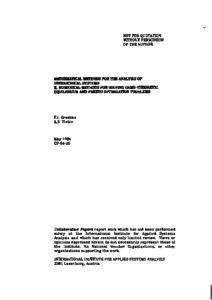# Mathematical Methods for the Analysis of Hierarchical Systems. II. Numerical Methods for Solving Game-Theoretic, Equilibrium and Pareto Optimization Problems

Ereshko, F. & Zlobin, A.S. (1984). Mathematical Methods for the Analysis of Hierarchical Systems. II. Numerical Methods for Solving Game-Theoretic, Equilibrium and Pareto Optimization Problems. IIASA Collaborative Paper. IIASA, Laxenburg, Austria: CP-84-020Preview
Text
CP-84-020.pdf

## Abstract

This is the second of two papers dealing with mathematical methods that can be used to analyze hierarchical systems. In this paper, the authors consider the case in which the lower level of a hierarchical system of decision makers is composed of a number of controllable subsystems. If these subsystems are not bounded by common constraints then the analysis is reduced to that of a two-level system consisting of a regulatory center and one lower subsystem. Two types of control are discussed in this case: control of resource use and control through price setting. If, however, there are shared resource-type constraints then it is assumed that the subsystems choose cooperatively from the set of Pareto-optimal alternatives. The problem for the regulatory center is then to maximize its goal function over this set. A number of ways of solving this problem are proposed, and a computational algorithm is given.

Item Type: Monograph (IIASA Collaborative Paper) Interactive Decision Analysis Program (IDA) IIASA Import 15 Jan 2016 01:55 27 Aug 2021 17:12 http://pure.iiasa.ac.at/2557View Item